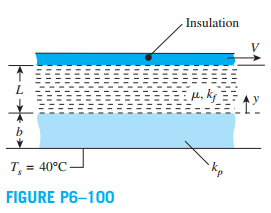Chat Now

# Consider the Couette flow of a fluid with a viscosity of µ 08 N sm2 and thermal conductivity of kf 0145 WmK The lower plate is stationary and made of a material of thermal conductivity

### Consider the Couette flow of a fluid with a viscosity of µ 08 N sm2 and thermal conductivity of kf 0145 WmK The lower plate is stationary and made of a material of thermal conductivity

Consider the Couette flow of a fluid with a viscosity of µ = 0.8 N · s/m2 and thermal conductivity of

kf = 0.145 W/m·K. The lower plate is stationary and made of a material of thermal conductivity kp = 1.5 W/m·K and thickness b = 3 mm. Its outer surface is maintained at Ts = 40°C. The upper plate is insulated and moves with a uniform speed V = 5 m/s. The distance between plates is L = 5 mm.

(a) Sketch the temperature distribution, T(y), in the fluid and in the stationary plate.

(b) Determine the temperature distribution function, T(y), in the fluid (0 < y=""><>

(c) Calculate the maximum temperature of the fluid, as well as the temperature of the fluid at the contact surfaces with the lower and upper plates.rahul 20-Jul-2020Get solution